Lakhmir Singh & Manjit Kaur: Gravitation, Solutions- 4

# Lakhmir Singh & Manjit Kaur: Gravitation, Solutions- 4 - Science Class 9

Page No -125

Question 46:
Explain why, buildings and dams have wide foundations.
Solution :
The foundations of buildings and dams are laid on a large area of ground so that the weight of the building or dam produces less pressure on the ground and they may not sink into the ground.

Question 47:
Why does a ship made of iron and steel float in water whereas a small piece of iron sinks in it ?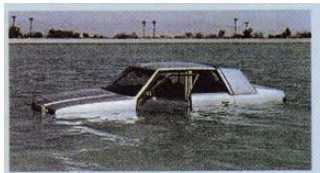The density of iron or steel is much higher than that of water, so an object made of iron or steel (like this car) sinks in water.

Solution :
A ship made of iron and steel is a hollow object which contains a lot of air in it. Due to the presence of a lot of air in it, the average density of the ship becomes less than the density of water. Hence a ship floats in water.
On the other hand, a piece of iron is denser than water, so it sinks in water.

Question 48:
Why do camels have large flat feet ?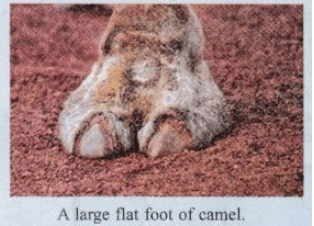Solution :
Camels have large flat feet so that there is a greater area in contact with the sand which produces less pressure on the sand and the camels can move easily on the sand.

Question 49:
Name these forces :
(a) the upward push of water on a submerged object
(b) the force which wears away two surfaces as they move over one another
(c) the force which pulled the apple off Isaac Newton’s tree.
(d) the force which stops you falling through the floor.

Solution :
(a) Buoyant force
(b) Force of friction
(c) Gravitational force
(d) Reaction force

Question 50:
A pressure of 10 Pa acts on an area of 3.0 m2. What is the force acting on the area ? What force will be exerted by the application of same pressure if the area is made one-third ?
Solution :
If the area is made one-third i.e. 1m2, then the force would be: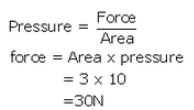If the area is made one-third i.e. 1m2, then the force would be: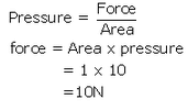Question 51:
A girl is wearing a pair of flat shoes. She weighs 550 N. The area of contact of one shoe with the ground is 160 cm2. What pressure will be exerted by the girl on the ground :
(a) if she stands on two feet ?
(b) if she stands on one foot ?
Solution :
Force, F= 550N
Area of contact of one shoe =160 cm2 =160 x 10-4m2
Area of contact with two shoes =160 x 2 =320 cm2=320 x 10-4m2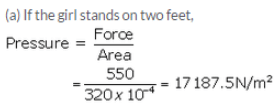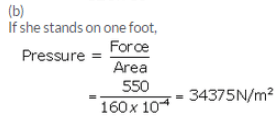Question 52:
Calculate the density of an object of volume 3 m3 and mass 9 kg. State whether this object will float or sink in water. Give reason for your answer.
Solution :

volume = 3 m3

Mass = 9Kg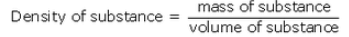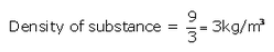and density of water = 1000kg/m
The object will float in the water as the density of the object is less than the density of water.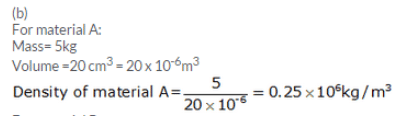Question 53:
An object weighs 500 grams in air. This object is then fully immersed in water. State whether it will weigh less in water or more in water. Give reason for your answer.
Solution :
The object will weigh less in water because an upward force (buoyant force) equal to the weight of water displaced acts on the object when immersed in water which reduces its weight apparently.

Question 54:
(a) Write down an equation that defines density.
(b) 5 kg of material A occupy 20 cm3 whereas 20 kg of materia! B occupy 90 cm3. Which has the greater density : A or B ? Support your answer with calculations.

Solution :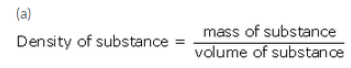For material B:
Mass =20kg
Volume =90 cm3 = 90x 10-6m3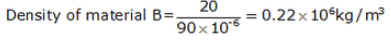Density of material A is more than density of material B.

Question 55:
(a) Define buoyant force. Name two factors on which buoyant force depends.
(b) What is the cause of buoyant force ?
(c) When a boat is partially immersed in water, it displaces 600 kg of water. How much is the buoyant force acting on the boat in newtons ? (g = 10 m s-2)

Solution :
(a) The upward force acting on an object immersed in a liquid is called buoyant force.
Factors affecting buoyant force:
(i) Volume of object immersed in the liquid,
(ii) Density of the liquid.
(b) The cause of buoyant force is the greater upward pressure exerted by water underneath the object..
(c) Mass of water displaced = 600kg
Weight of water displaced, W =m x g
=600 x 10 =6000N
Since, the weight of water displaced by the boat is 6000N, therefore the buoyant force acting on the boat will also be 6000N.

Question 56:
(a) State the principle of flotation.
(b) A floating boat displaces water weighing 6000 newtons.
(i) What is the buoyant force on the boat ?
(ii) What is the weight of the boat ?

Solution :
(a) According to the principle of floatation: An object will float in a liquid if the weight of object is equal to the weight of liquid displaced by it.
Weight of object = Weight of liquid displaced by it.
(b) Weight of water displaced by boat= 6000N
(i) Buoyant force =6000N, as the weight of water displaced is equal to buoyant force.
(ii) Weight of a floating object = Weight of water displaced by it = 6000N

Question 58:
(a) Define pressure.
(b) What is the relation between pressure, force and area ? ,
(c) Calculate the pressure when a force of 200 N is exerted on an area of:
(i) 10 m2
(ii) 5 m
2
Solution :
(a) Pressure is the force acting perpendicularly on a unit area of the object.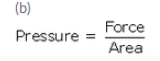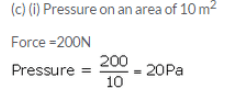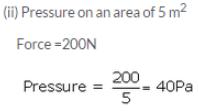Page No -126

Question 59:
(a) What are fluids ? Name two common fluids.
(b) State Archimedes’ principle.
(c) When does an object float or sink when placed on the surface of a liquid ?

Solution :
(a) Those substances which can flow easily are called fluids. All the liquid and gases are fluids, like water, air etc.
(b) Archimedes’ Principle :
When an object is wholly (or partially) immersed in a liquid, it experiences a buoyant force (or upthrust) which is equal to the weight of liquid displaced by the object.
Buoyant force on an object = weight of liquid displaced by that object
(c) If the buoyant force exerted by the liquid is less than the weight of the object, the object will sink in the liquid. If the buoyant force exerted by the liquid is equal to or greater than the weight of the object, the object will float in the liquid.

Question 60:
(a) How does a boat float in water ?
(b) A piece of steel has a volume of 12 cm3, and a mass of 96 g. What is its density :
(i) in g/cm3 ?
(ii) in kg/m3 ?

Solution :
(a) A floating boat displaces water equal to its own weight. This displaced water exerts buoyant force to balance the weight of boat and keep it afloat.
(b) (i) Mass = 96 g
Volume = 12cm3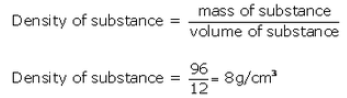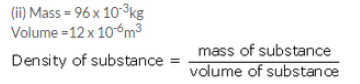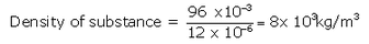Question 61:
An elephant weighing 40,000 N stands on one foot of area 1000 cm2 whereas a girl weighing 400 N is standing on one ‘stiletto’ heel of area 1 cm2.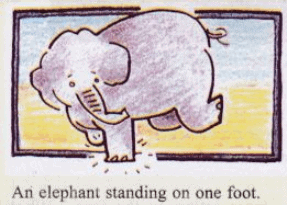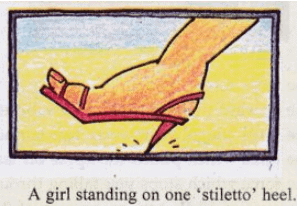(a) Which of the two, elephant or girl, exerts a larger force on the ground and by how much ?
(b) What pressure is exerted on the ground by the elephant standing on one foot ?
(c) What pressure is exerted on the ground by the girl standing on one heel ?
(d) Which of the two exerts larger pressure on the ground : elephant or girl ?
(e) What is the ratio of pressure exerted by the girl to the pressure exerted by the elephant ?
Solution :
Weight of elephant=40000N
Area of one foot =1000 cm2= 1000 x 10-4m2
Weight of girl=400N
Area of heel of girl =1 cm2=1 x 10-4m2
(a) Elephant has a larger weight of 40000N, therefore, elephant exerts a larger force on the ground. Elephant exerts a larger force on the ground by 40000N – 400 N=39600N.
(b)Weight of elephant = 40000N
Area of one foot =1000cm2= 1000 x 10-4m2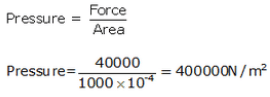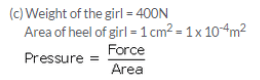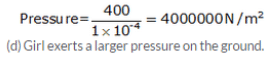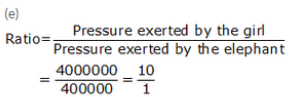The pressure exerted by girl is 10 times greater than the exerted by the elephant.

Page No -127

Question 72:
If two equal weights of unequal volumes are balanced in air, what will happen when they are completely dipped in water ? Why ?
Solution :
The two equal weights of unequal volumes which are balanced in air, will get imbalanced when they are completely dipped in water because due to their unequal volumes, they will displace unequal volumes of water and hence suffer unequal loss in weight.

Question 73:
Two different bodies are completely immersed in water and undergo the same loss in weight. Is it necessary that their weights in air should also be the same ? Explain.
Solution :
No, it is not necessary that their weights in air should also be the same. This is because the two bodies have undergone the same loss in weight on completely immersing in water due to their equal volumes and not because of their equal weights, so they may have different weights in air.

Question 74:
A body floats in kerosene of density 0.8 x 103 kg/m3 up to a certain mark. If the same body is placed in water of density 1.0 x 103 kg/m3, will it sink more or less ? Give reason for your answer.
Solution :
The body will sink less in water. This is because the density of water is more than that of kerosene due to which water will exert a greater upward buoyant force on the body.

Question 75:
Giving reasons state the reading on a spring balance when it is attached to a floating block of wood which weighs 50 g in air.
Solution :
The reading on spring balance will be zero. This is because the weight of floating block of wood is fully supported by the liquid in which it is floating and hence it does not exert any force on the spring balance.

Question 76:
If a fresh egg is put into a beaker filled with water, it sinks. On dissolving a lot of salt in the water, the egg begins to rise and then floats. Why ?
Solution :
When a lot of salt is dissolved in water, then the density of salt solution becomes much more than pure water. Due to its much higher density, the salt solution exerts a greater upward buoyant force on the egg making it rise and then float.

Page No:128

Question 77:
A beaker full of water is suspended from a spring balance. Will the reading of the balance change :
(a) if a cork is placed in water ?
(b) if a piece of heavy metal is placed in it ?

Solution :
The reading of spring balance will not change if a cork is placed in water because cork, being lighter than water, floats in water.
(b)The reading of spring balance will change if a piece of heavy metal is placed in
water because heavy metal being denser than water, sinks in water.

Question 78:
When a golf ball is lowered into a measuring cylinder containing water, the water level rises by 30 cm3 when the ball is completely submerged. If the mass of ball in air is 33 g, find its density.
Solution :
Volume of golf ball = rise in water level = 30 cm3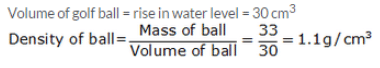Question 79:

A boy gets into a floating boat.

• What happens to the boat ?
• What happens to the weight of water displaced ?
• What happens to the buoyant force on the boat ?

Solution :
a) The boat sinks a little more in water, that is, the boat floats lower in water.
b) The weight of water displaced (by the submerged part of the boat) increases.
c) The buoyant force acting on the boat increases.

Question 80:
A y kg sheet of tin sinks in water but if the same sheet is converted into a box or boat, it floats. Why ?
Solution :
The sheet of tin sinks in water because the density of tin is higher than that of water. When the same sheet of tin is converted into a box or a boat, then due to the trapping of lot of ‘light’ air in the box or boat, the average density of the box or boat made of tin sheet becomes lower than that of water and hence it floats in water.

The document Lakhmir Singh & Manjit Kaur: Gravitation, Solutions- 4 | Science Class 9 is a part of the Class 9 Course Science Class 9.
All you need of Class 9 at this link: Class 9

## Science Class 9

66 videos|355 docs|97 tests

## FAQs on Lakhmir Singh & Manjit Kaur: Gravitation, Solutions- 4 - Science Class 9

 1. What is the definition of gravitation?Ans. Gravitation is the force of attraction that exists between any two objects with mass. It is responsible for the movement of planets, the formation of galaxies, and the overall structure of the universe.
 2. How does the force of gravitation work?Ans. The force of gravitation works based on the principle of mass attracting mass. Every object with mass exerts a gravitational force on other objects around it. The magnitude of the force depends on the masses of the objects and the distance between them. The force of gravitation always acts towards the center of the objects.
 3. What is the universal law of gravitation?Ans. The universal law of gravitation, formulated by Sir Isaac Newton, states that every particle in the universe attracts every other particle with a force that is directly proportional to the product of their masses and inversely proportional to the square of the distance between them. This law mathematically represents the force of gravitation.
 4. What are the applications of gravitation in everyday life?Ans. Gravitation has numerous applications in our daily lives. Some examples include: - The movement of tides in oceans and seas due to the gravitational pull of the moon and the sun. - The functioning of various tools and instruments such as weighing scales, spring balances, and accelerometers, which rely on gravity for their accurate measurements. - The orbiting of satellites around the Earth and their navigation systems, which are based on the principles of gravitation. - The calculation of the weight of objects and the determination of their mass using the formula F = mg, where F is the force of gravity and g is the acceleration due to gravity.
 5. How does gravitation affect the motion of planets?Ans. Gravitation is responsible for the motion of planets in their orbits around the sun. According to Newton's laws of motion and the law of gravitation, the gravitational force exerted by the sun on a planet provides the centripetal force required to keep the planet in a stable orbit. The planet's motion is a balance between the gravitational force pulling it towards the sun and its own inertia trying to carry it in a straight line. This results in a continuous elliptical orbit around the sun.

## Science Class 9

66 videos|355 docs|97 testsExplore Courses for Class 9 examSignup to see your scores go up within 7 days! Learn & Practice with 1000+ FREE Notes, Videos & Tests.
10M+ students study on EduRev
Track your progress, build streaks, highlight & save important lessons and more!
Related Searches

,

,

,

,

,

,

,

,

,

,

,

,

,

,

,

,

,

,

,

,

,

,

,

,

;